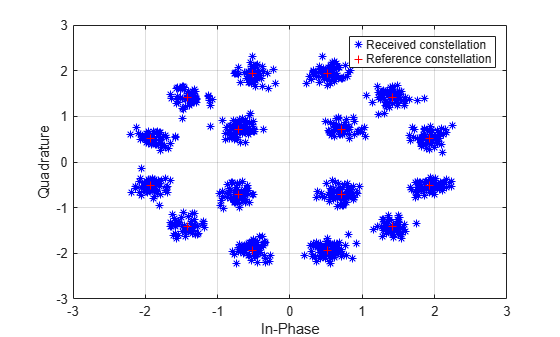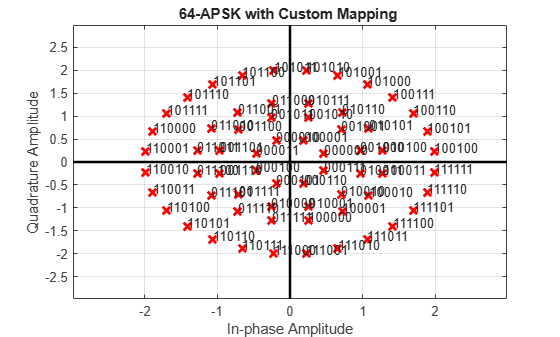# apskdemod

Amplitude phase shift keying (APSK) demodulation

## Syntax

``z = apskdemod(y,M,radii)``
``z = apskdemod(y,M,radii,phaseoffset)``
``z = apskdemod(___,Name,Value)``

## Description

example

````z = apskdemod(y,M,radii)` performs APSK demodulation of the input signal `y`, based on the specified number of constellation points per PSK ring, `M`, and the radius of each PSK ring, `radii`. For a description of APSK demodulation, see APSK Hard Demodulation and APSK Soft Demodulation. Noteapskdemod specifically applies to multiple ring PSK constellations. For a single ring PSK constellation, use `pskdemod`. ```
````z = apskdemod(y,M,radii,phaseoffset)` specifies an initial phase offset for each PSK ring of the APSK modulated signal.```

example

````z = apskdemod(___,Name,Value)` specifies options using one or more name-value pair arguments using any of the previous syntaxes. For example, `'OutputDataType','double'` specifies the desired output data type as double. Specify name-value pair arguments after all other input arguments.```

## Examples

collapse all

Demodulate a 16-APSK signal that has an unequal number of constellation points on each circle. Plot the received constellation.

Define vectors for modulation order and PSK ring radii. Generate random 16-ary data symbols.

```M = [4 12]; radii = [1 2]; modOrder = sum(M); x = randi([0 modOrder-1],1000,1);```

Apply APSK modulation to the data.

`txSig = apskmod(x,M,radii);`

Pass the modulated signal through a noisy channel.

```snr = 20; % dB rxSig = awgn(txSig,snr,'measured');```

Plot the transmitted (reference) signal points and the noisy received signal points.

```plot(rxSig,'b*') hold on grid plot(txSig,'r+') xlim([-3 3]) ylim([-3 3]) xlabel('In-Phase') ylabel('Quadrature') legend('Received constellation','Reference constellation')```Demodulate the received signal and compare to the input data.

```z = apskdemod(rxSig,M,radii); isequal(x,z)```
```ans = logical 1 ```

Demodulate a 64-APSK signal with custom symbol mapping. Compute hard decision bit output and verify that the input matches the output.

Define vectors for modulation order and PSK ring radii. Generate 100 symbols of random bit input.

```M = [8 12 16 28]; % 4-PSK circles modOrder = sum(M); radii = [0.5 1 1.3 2]; x = randi([0 1],100*log2(modOrder),1);```

Create a custom symbol mapping vector of binary mapping.

`cmap = 0:63; `

Modulate the data and plot the constellation.

```y = apskmod(x,M,radii,'SymbolMapping',cmap,'inputType','bit', ... 'PlotConstellation',true);````z = apskdemod(y,M,radii,'SymbolMapping',cmap,'OutputType','bit');`

Verify that the demodulated signal is equal to the original data.

`isequal(x,z)`
```ans = logical 1 ```

Demodulate a 32-APSK signal and calculate soft bits.

Define vectors for modulation order and PSK ring radii. Generate 10000 symbols of random bit data.

```M = [16 16]; modOrder = sum(M); radii = [0.6 1.2]; numSym = 10000; x = randi([0 1], numSym*log2(modOrder),1); ```

Generate a reference constellation. Create a constellation diagram object.

```refAPSK = apskmod(0:modOrder-1,M,radii); constDiagAPSK = comm.ConstellationDiagram('ReferenceConstellation',refAPSK, ... 'Title','Received Symbols','XLimits',[-2 2],'YLimits',[-2 2]); ```

Modulate the data.

```txSig = apskmod(x,M,radii,'InputType','bit'); sigPow = var(txSig); ```

Pass the signal through a noisy channel.

```snr = 15; rxSig = awgn(txSig,snr,sigPow,'linear'); ```

Plot the reference and received constellation symbols.

```constDiagAPSK(rxSig) ```Demodulate the signal and compute soft bits.

```z = apskdemod(rxSig,M,radii,'OutputType','approxllr', ... 'NoiseVariance',sigPow/snr); ```

## Input Arguments

collapse all

APSK modulated signal, specified as a complex scalar, vector, or matrix. Each column is treated as an independent channel.

Data Types: `double` | `single`
Complex Number Support: Yes

Constellation points per PSK ring, specified as a vector with more than one element. Vector elements indicate the number of constellation points in each PSK ring. The first element corresponds to the innermost circle, and so on, until the last element, that corresponds to the outermost circle. Element values must be multiples of four and `sum(M)` must be a power of two. The modulation order is the total number of points in the signal constellation and equals the sum of the vector elements, `sum(M)`.

Example: `[4 12 16]` specifies a three PSK ring constellation with a modulation order of `sum(M)` = 32.

Data Types: `double`

PSK ring radii, specified as a vector with the same length as `M`. The first element corresponds to the innermost circle, and so on, until the last element, that corresponds to the outermost circle. The elements must be positive and arranged in increasing order.

Example: `[0.5 1 2]` defines constellation PSK ring radii. The inner ring has a radius of 0.5, the second ring has a radius of 1.0, and the outer ring has a radius of 2.0.

Data Types: `double`

Phase offset of each PSK ring in radians, specified as a scalar or vector with the same length as `M`. The first element corresponds to the innermost circle, and so on, until the last element, that corresponds to the outermost circle. The `phaseoffset` can be a scalar only if all the elements of `M` are the same value.

Example: `[pi/4 pi/12 pi/16]` defines three constellation PSK ring phase offsets. The inner ring has a phase offset of pi/4, the second ring has a phase offset of pi/12, and the outer ring has a phase offset of pi/16.

Data Types: `double`

### Name-Value Pair Arguments

Specify optional comma-separated pairs of `Name,Value` arguments. `Name` is the argument name and `Value` is the corresponding value. `Name` must appear inside quotes. You can specify several name and value pair arguments in any order as `Name1,Value1,...,NameN,ValueN`.

Example: ```y = apskdemod(x,M,radii,'OutputType','bit','OutputDataType','single');```

Symbol mapping, specified as the comma-separated pair consisting of `SymbolMapping` and one of the following:

• `'contourwise-gray'` — Uses Gray mapping along the contour in phase dimension.

• `'gray'` — Uses Gray mapping along the contour in both the amplitude and phase dimensions. For Gray symbol mapping, all the values for `M` must be equal and all the values for `phaseoffset` must be equal. For a description of the Gray mapping used, see .

• integer vector — Use custom symbol mapping. Vector must consist of `sum(M)` unique elements with values from 0 to (`sum(M)-1`). The first element corresponds to the constellation point in the first quadrant of the innermost circle, with subsequent elements positioned counterclockwise around the PSK rings.

The default symbol mapping depends on `M` and `phaseOffset`. When all the elements of `M` and `phaseOffset` are equal, the default is `'gray'`. For all other cases, the default is `'contourwise-gray'`.

Data Types: `double` | `char` | `string`

Output type, specified as the comma-separated pair consisting of `'OutputType'` and `'integer'`, `'bit'`, `'llr'`, or `'approxllr'`. For a description of the returned output, see `z`.

Data Types: `char` | `string`

Output data type, specified as the comma-separated pair consisting of `OutputDataType` and one of the indicated data types. Acceptable values for `OutputDataType` depend on the `OutputType` value.

`OutputType` ValueAcceptable `OutputDataType` Values
`'integer'``'double'`, `'single'`, `'int8'`, `'int16'`, `'int32'`, `'uint8'`, `'uint16'`, or `'uint32'`
`'bit'``'double'`, `'single'`, `'int8'`, `'int16'`, `'int32'`, `'uint8'`, `'uint16'`, `'uint32'`, or `'logical'`

#### Dependencies

This name-value pair argument applies only when `OutputType` is set to `'integer'` or `'bit'`.

Data Types: `char` | `string`

Noise variance, specified as the comma-separated pair consisting of `NoiseVariance` and a positive scalar or vector of positive values.

• When specified as a scalar, the same noise variance value is used on all input elements.

• When specified as a vector, the vector length must be equal to the number of columns in the input signal.

When the noise variance or signal power result in computations involving extreme positive or negative magnitudes, see APSK Soft Demodulation for algorithm selection considerations.

#### Dependencies

This name-value pair argument applies only when `OutputType` is set to `'llr'` or `'approxllr'`.

Data Types: `double`

Option to plot constellation, specified as the comma-separated pair consisting of `'PlotConstellation'` and a logical scalar. To plot the constellation, set `PlotConstellation` to `true`.

Data Types: `logical`

## Output Arguments

collapse all

Demodulated signal, returned as a scalar, vector, or matrix. The dimensions of `z` depend on the specified `OutputType` value.

`OutputType` ValueReturn Value of `apskdemod`Dimensions of `z`
`'integer'`Demodulated integer values from 0 to (sum(`M`) – 1)`z` has the same dimensions as input `y`.
`'bit'`Demodulated bitsThe number of rows in `z` is log2(sum(`M`)) times the number of rows in `y`. Each demodulated symbol is mapped to a group of log2(sum(`M`)) elements in a column, where the first element represents the MSB and the last element represents the LSB.
`'llr'`Log-likelihood ratio value for each bit
`'approxllr'`Approximate log-likelihood ratio value for each bit

collapse all

### APSK Hard Demodulation

The hard demodulation algorithm applies amplitude phase decoding as described in .### APSK Soft Demodulation

For soft demodulation, two soft-decision log-likelihood ratio (LLR) algorithms are available: exact LLR and approximate LLR. This table compares these algorithms.

AlgorithmAccuracyExecution Speed
Exact LLRmore accurateslower execution
Approximate LLRless accuratefaster execution

For further description of these algorithms, see Exact LLR Algorithm and Approximate LLR Algorithm.

### Note

The exact LLR algorithm computes exponentials using finite precision arithmetic. Computation of exponentials with very large positive or negative magnitudes might yield:

• `Inf` or `-Inf` if the noise variance is a very large value

• `NaN` if both the noise variance and signal power are very small values

When the output returns any of these values, try using the approximate LLR algorithm because it does not compute exponentials.

 Sebesta, J. “Efficient Method for APSK Demodulation.” Selected Topics on Applied Mathematics, Circuits, Systems, and Signals (P. Pardalos, N. Mastorakis, V. Mladenov, and Z. Bojkovic, eds.). Vouliagmeni, Athens, Greece: WSEAS Press, 2009.

 Liu, Z., Q. Xie, K. Peng, and Z. Yang. "APSK Constellation with Gray Mapping." IEEE Communications Letters. Vol. 15, Number 12, December 2011, pp. 1271–1273.